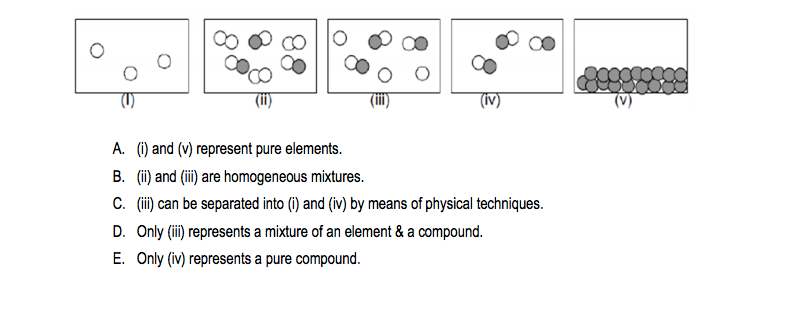Problem: These figures represent examples of matter and its composition. Which statement is INCORRECT?

FREE Expert Solution
81% (258 ratings)
Problem Details

These figures represent examples of matter and its composition. Which statement is INCORRECT?What scientific concept do you need to know in order to solve this problem?

Our tutors have indicated that to solve this problem you will need to apply the Classification of Matter concept. You can view video lessons to learn Classification of Matter Or if you need more Classification of Matter practice, you can also practice Classification of Matter practice problems .

What is the difficulty of this problem?

Our tutors rated the difficulty of These figures represent examples of matter and its compositi... as low difficulty.

How long does this problem take to solve?

Our expert Chemistry tutor, Jules took 4 minutes to solve this problem. You can follow their steps in the video explanation above.

What professor is this problem relevant for?

Based on our data, we think this problem is relevant for Professor Brydges' class at UCSD.